# What Is WACC? (+How Companies, Investors, And You Can Use It)

If you know the first thing about capital structure, you know that companies fund themselves with a combination of debt and equity.

A company’s capital structure is defined by how much debt and how much equity makes up its total capital used to purchase assets, fund operations and growth, and more—but how does a company decide on an optimal mix? Weighted average cost of capital, or WACC can help.

# What is WACC?

A company’s WACC is a calculation of the cost of all of its capital, or the money it uses to purchase assets.

All capital, both debt capital and equity capital, comes at a cost—but each source of funding is unique in how much it will end up costing the company over time, and how much of the company’s total capital it makes up.

The WACC formula produces the sum of the cost of capital of each funding source, amounting to the total cost of capital for a company. That means accounting for the individual cost of a company’s common and/or preferred stock, loans, bonds, and more, then weighting each cost accordingly.

This way, companies determine how optimal their capital structure is, and investors determine whether the company is stable enough to invest in. It also helps determine the enterprise value of a company, or what the company is worth.

The formula below is commonly used to find WACC. Don’t do formulas? Excel can do the work for you in our free calculator.

E = company equity

D = company debt

V = total value of company capital

E/V = percentage equity

D/V = percentage debt

Re = cost of equity

Rd = cost of debt

T = tax rate

You find WACC by multiplying a company’s cost of equity by the percentage of equity financing (or how much of the capital structure is made up of equity), then adding that to the cost of debt multiplied by the percentage of debt and one minus the tax rate. If the company has also issued preferred stock, you’ll need to calculate the cost of preferred stock, then multiply that by the percentage of preferred stock.

 Tip: Debt financing is not taxed, but equity is, which is why the tax rate is factored into the debt side of the equation and not the equity side.

Still confused? It’s not just you—this equation is complex! There is a lot of information needed to calculate WACC. One thing you can do to help make sense of WACC is make sure you have an understanding of how to calculate cost of of equity using the capital asset pricing model (CAPM), as well as how to calculate cost of debt. You’ll need to make those calculations first, then you can plug your results into the WACC equation.

## WACC example

We’ll break down how to calculate WACC using Coca Cola as an example, but WACC is typically calculated using an online calculator or excel formula. You can download our free Excel template to make the calculation easier.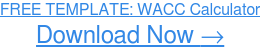### How to calculate WACC using the formula

The first step in calculating WACC using the formula is finding the company's cost of debt and cost of equity. For this example, we’re using Coca Cola. Because it is a large, publicly-traded corporation, information on Coca Cola’s finances is readily available on the internet. Google it, or use a source like Nasdaq to find this data. Here is the information we’ll be using:

Coca Cola (KO)

Interest expense: \$919,000,000

Total liabilities: \$66,235,000,000

Total equity: \$16,981,000,000

Total capital: \$83,981,000,000

Tax rate: 21%

Beta: 0.48

Calculating WACC also requires some general information on the market, all of which can also be found on the internet. Find the market risk by subtracting the risk-free rate from the expected return on investment in the stock market:

Risk-free rate: 2.17%

Market risk: 7.83%

 Tip: Your answer might differ if you use different values for the risk-free rate and market risk, but that’s okay! It does not make your answer less correct.

First, we calculated Coca Cola’s cost of debt using the formula below: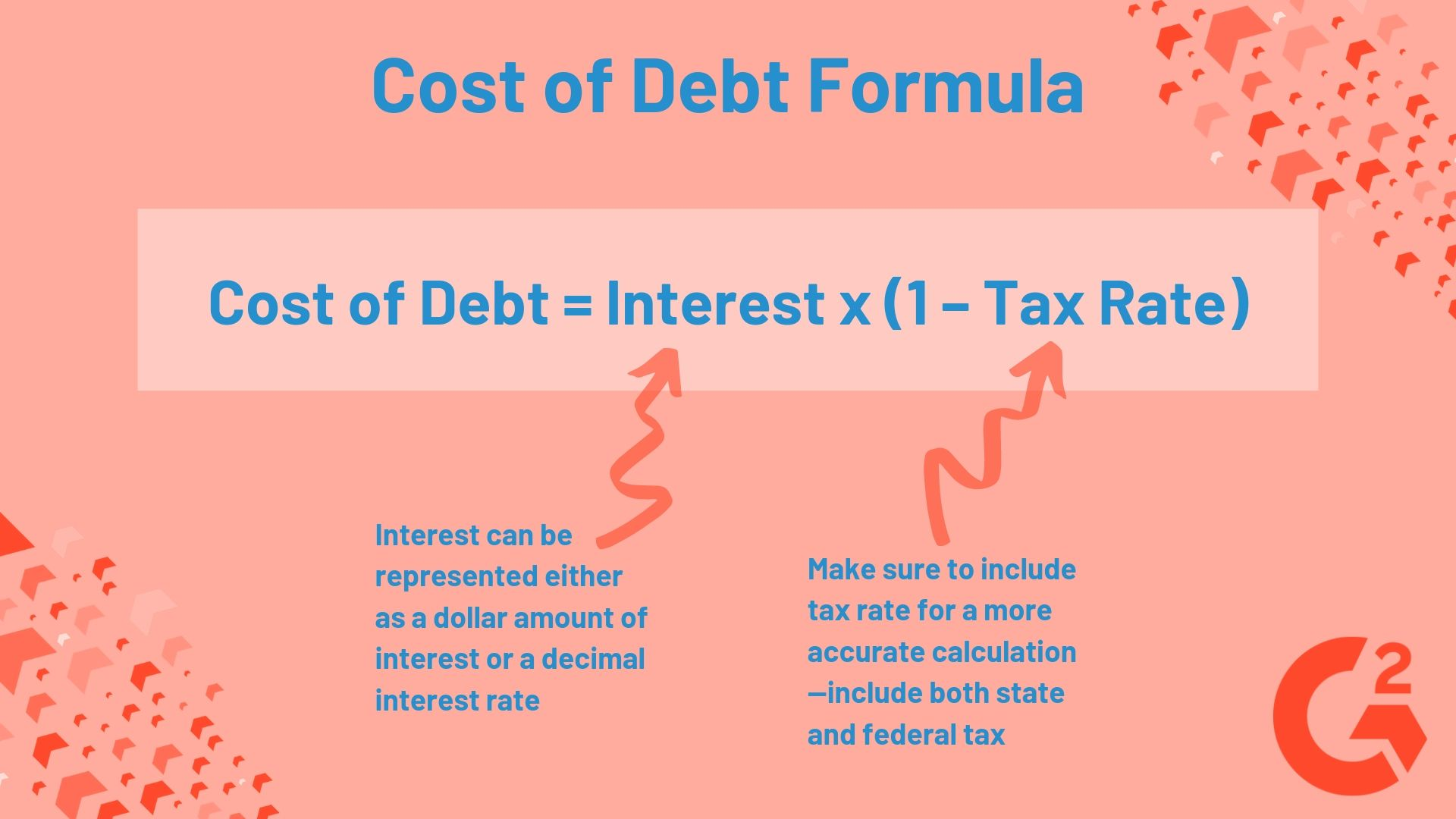Tip: find the interest rate by dividing the company’s interest expense by its total liabilities. For Coca Cola, divide \$19,000,000 / \$66,235,000,000 to get 0.014, or 1.4%.

Cost of debt = 0.014 (1 - 0.21) = 0.011

Coca Cola’s cost of debt is 0.011.

Next, let’s calculate Coca Cola’s cost of equity using the capital asset pricing model.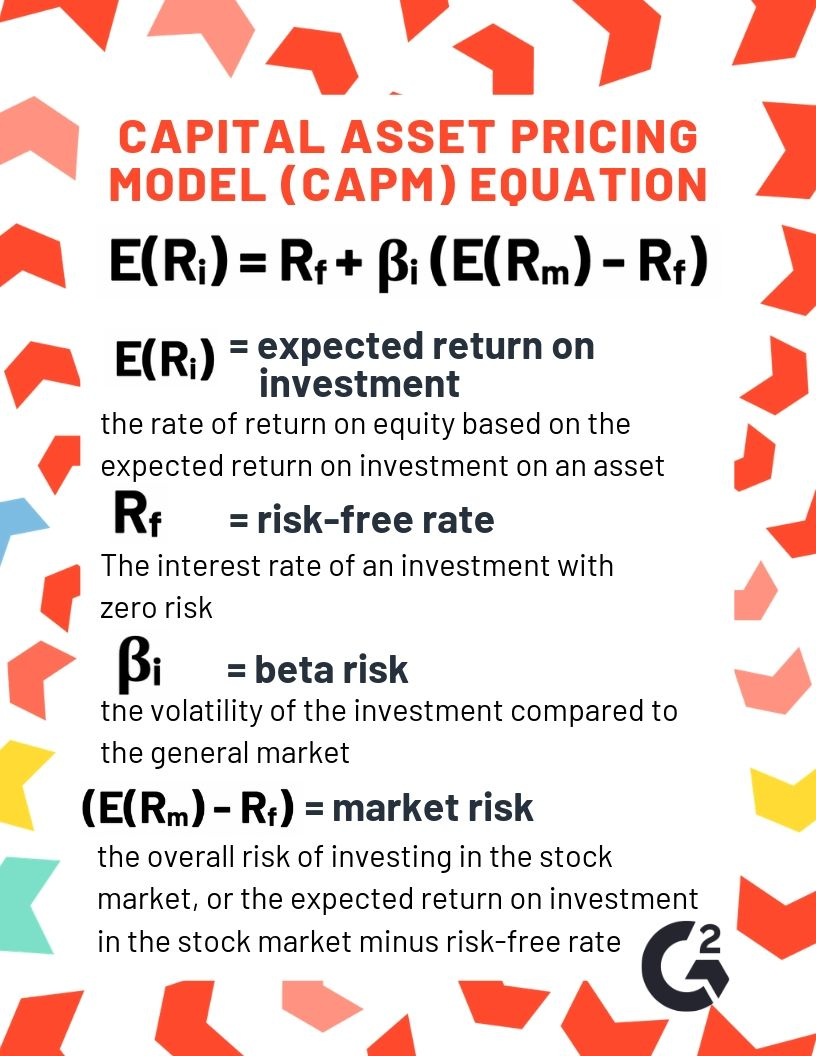Cost of equity = 0.0217 + 0.48(0.0783) = 0.059

Coca Cola’s cost of equity is 0.059.

Before we can put it all together and find Coca Cola’s WACC, we have to figure out how much of its capital structure is debt, and how much is equity. This is important, as it will determine the “weight” of each source of company capital. We can calculate this by dividing total equity by total capital, then doing the same for debt (represented as total liabilities on a company balance sheet). Like so:

% equity = \$16,981,000,000 / \$83,981,000,000 = 0.2041 or 20.41%

% debt = \$66,235,000,000 / \$83,981,000,000 = 0.7959 or 79.59%

 Tip: When you total the decimals or percentages for each cost of capital, you should get 1 or 100%.

You’re almost there! The last step is plugging all of these values into the WACC equation:

WACC = (0.059 x 0.2041) + (0.011 x .7959 x (1- 0.21)) = 0.019 or 1.9%

That’s a pretty good WACC, don’t you think? Especially when you consider the industry average is 8.50%.

### How to calculate WACC in excel

If you thought that was tedious, you’re not wrong. Calculating WACC with a pen and paper might help you get a better understanding of it, but if you want to experiment with different hypothetical values or calculate the WACC of multiple companies quickly, you’re going to need something a bit more powerful. Download the excel template above, then all you have to do is enter accurate values, and let the excel formula calculate the WACC for you.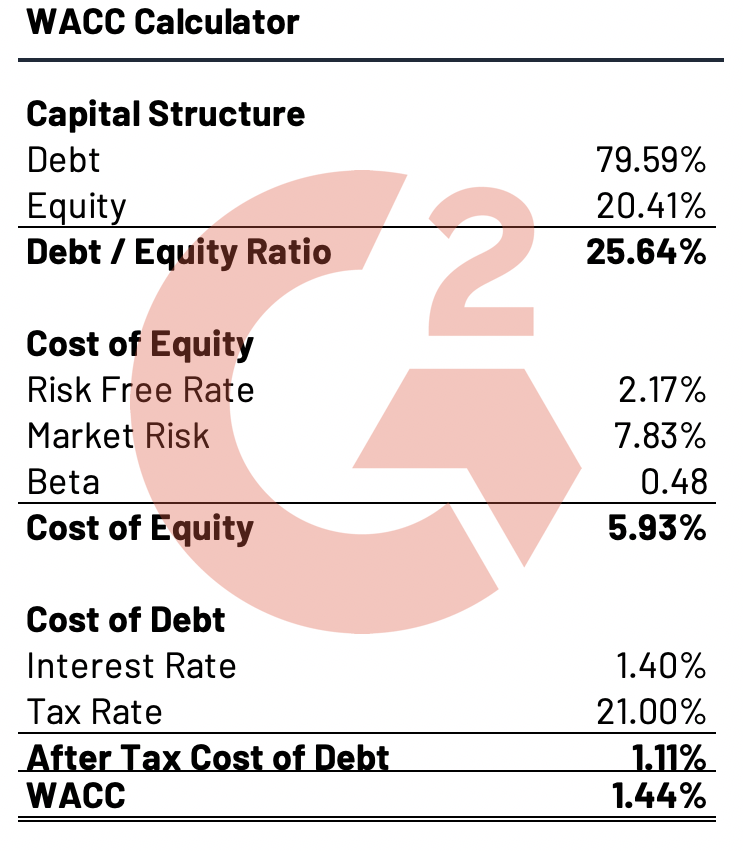Tip: Not only is it faster to calculate WACC in Excel, but it will also produce more accurate results down to the last decimal.

If all of this sounds too complicated, there are still other alternatives you can use. Save yourself time and headache by utilizing financial analysis software to calculate WACC and take a more in-depth look at your company’s finances.

Financial analysis software can help your business consolidate financial data from multiple systems, generate reports that comply with IFRS and GAAP standards and perform complicated calculations like the ones we’ve seen above with ease.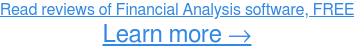#### WACC yourself silly

You now have everything you need to calculate WACC. Experiment and see what you can find out about companies’ capital structure using your knowledge, our template, or both!